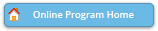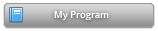#### Abstract Details

 Activity Number: 548 - Total Survey Errors in the Combination of Probability and Nonprobability Samples Type: Invited Date/Time: Wednesday, July 31, 2019 : 2:00 PM to 3:50 PM Sponsor: Survey Research Methods Section Abstract #300113 Presentation Title: Combining Data from a Probability and Nonprobability Sample Using a Composite Estimator Author(s): Burton Levine* Companies: RTI International Keywords: nonprobability; composite estimator; redirected inbound call sampling Abstract: We present methodology for combining probability and nonprobability samples that could improve the quality of survey estimates. Extending Elliott and Haviland’s (2007) results, we develop an optimal composite estimator that accommodates the scenario where both the probability and nonprobability estimates contain known bias in an estimate. Using our derived composite estimator, we examine the relationship between the bias characteristics of the samples and the weight attributed to each sample by the compositing factor. If the nonprobability sample estimate has much more bias than the probability sample, the composite estimator is mostly a function of the probability data. We present methodology to quantify an allocation of probability and nonprobability sample that minimizes the mean squared error of the estimate based on cost per completed interview and the bias and variance characteristics of estimates from the two samples. The addition of cost to the analysis will inform future allocations of probability and nonprobability respondents. We illustrate the application of these methods to combine a probability telephone sample and a nonprobability redirected inbound call sample.

Authors who are presenting talks have a * after their name.